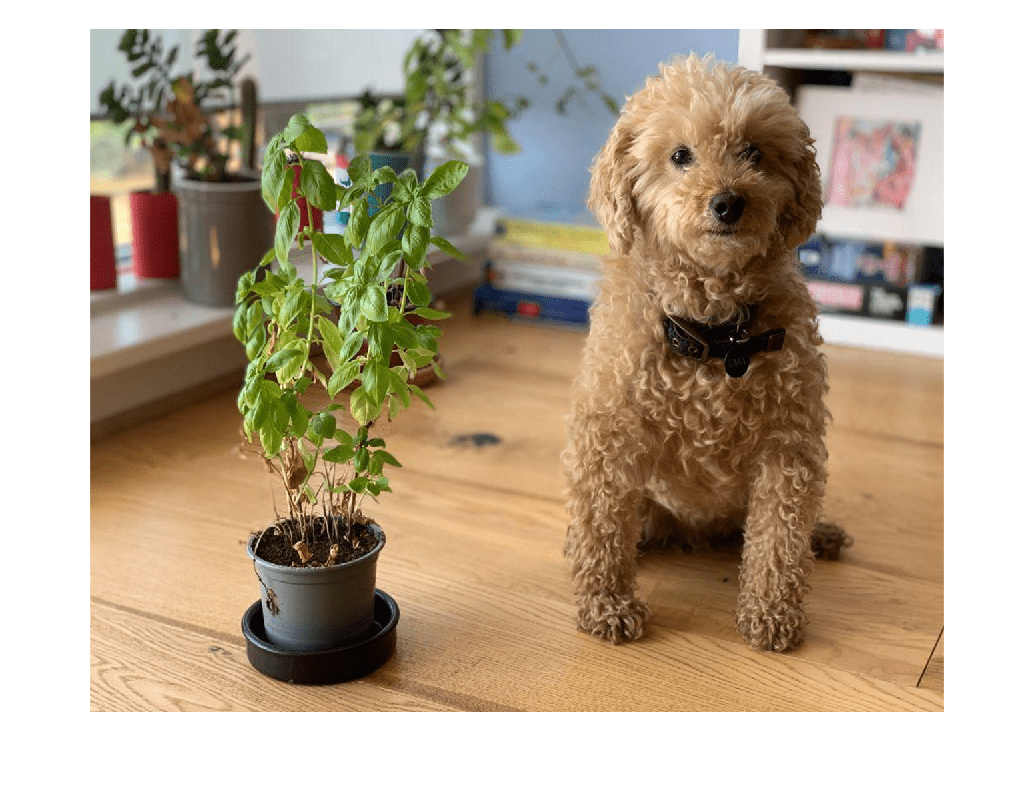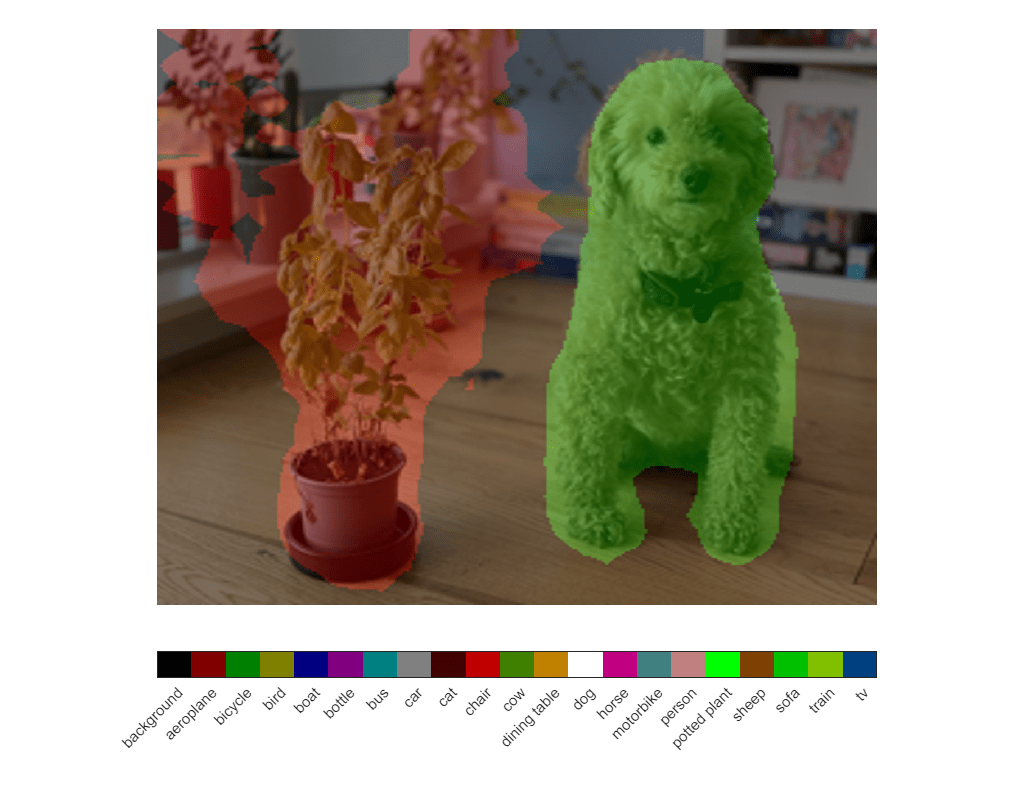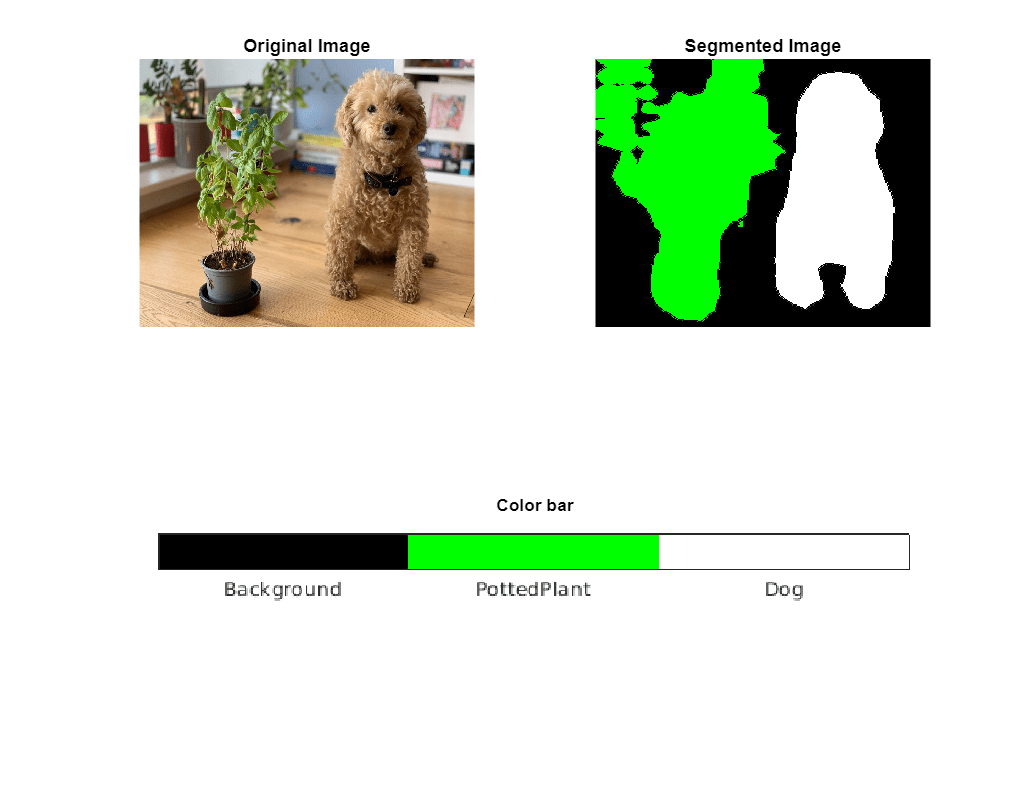# Deploy Semantic Segmentation Application Using TensorFlow Lite Model on Host and Raspberry Pi

This example shows how to simulate and generate code for an image segmentation application that uses a Tensorflow Lite(TFLite) model. This example uses DeepLab V3 TensorFlow Lite model from the TensorFlow hub. This model can segment each pixel of the input image into 21 classes, such as background, dog, and plant.

### Third-Party Prerequisites

• Raspberry Pi hardware

• TFLite library on the target ARM® hardware

This example uses TFLites to run inference on a pretrained DeepLab V3 model.

Run these commands to download the DeepLab V3 model.The model file is approximately 2.7 MB in size.

```if ~exist("deeplabv3.tflite","file") disp('Downloading Deeplabv3 model file...'); url = "https://tfhub.dev/tensorflow/lite-model/deeplabv3/1/metadata/2?lite-format=tflite"; websave("deeplabv3.tflite",url); end```

### The Entry-Point Function

The `tflite_semantic_predict` entry-point function loads the DeepLab V3 model into a persistent network object using the `loadTFLiteModel` function. Then, the function performs prediction by passing the network object to the `predict` function.

`type tflite_semantic_predict.m`
```function output = tflite_semantic_predict(in) persistent net; if isempty(net) net = loadTFLiteModel('deeplabv3.tflite'); end output= net.predict(in); end ```

The `loadTFLiteModel` function creates a `TFLiteModel` object. `TFLiteModel` object has properties that contain information about the model, such as the number and size of inputs and outputs of the model. For more information on `loadTFLiteModel` (Deep Learning Toolbox) and `loadTFLiteModel` (Deep Learning Toolbox).

```net = loadTFLiteModel('deeplabv3.tflite'); disp(net);```
``` TFLiteModel with properties: ModelName: 'deeplabv3.tflite' NumInputs: 1 NumOutputs: 1 InputSize: {[257 257 3]} OutputSize: {[257 257 21]} InputScale: 1 InputZeroPoint: 0 OutputScale: 1 OutputZeroPoint: 0 InputType: {["FP32"]} OutputType: {["FP32"]} NumThreads: 6 Mean: 127.5000 StandardDeviation: 127.5000 ```

Read the labels file associated with TFLite Model.

`labelsFile = importdata('labelmap_segmentation.txt');`

### Perform Semantic Segmentation by Using Simulation on Host

Load an input image and call `tflite_semantic_predict` on the input image.

```I = imread("testMultilabelImage2.png"); I1 = imresize(I,[257 257]); I2 = imresize(I,[1024,1280]); imshow(I2);````output = tflite_semantic_predict(I1);`
``` TFLiteModel with properties: ModelName: 'deeplabv3.tflite' NumInputs: 1 NumOutputs: 1 InputSize: {[257 257 3]} OutputSize: {[257 257 21]} InputScale: 1 InputZeroPoint: 0 OutputScale: 1 OutputZeroPoint: 0 InputType: {["FP32"]} OutputType: {["FP32"]} NumThreads: 6 Mean: 127.5000 StandardDeviation: 127.5000 ```

### Generate MEX for the `tflite_semantic_predict` Function

Use the `codegen` command to generate a MEX function that runs on the host platform.

Create a code configuration object for a MEX function and set the target language to C++. To generate MEX, use the `codegen` command and specify the input size as [257,257,3]. This value corresponds to the input layer size of the network.

```cfg = coder.config('mex'); cfg.TargetLang = 'C++'; codegen -config cfg tflite_semantic_predict -args ones(257,257,3,'single')```
```Code generation successful. ```

Call `tflite_semantic_predict_mex` on the input image `I1`.

`output = tflite_semantic_predict_mex(single(I1));`

### Generate, Deploy and Run Code on Raspberry Pi

On the Raspberry Pi hardware, set the environment variable `TFLITE_PATH` to the location of the TFLite library. For more information on how to build the TFLite library and set the environment variables, see Prerequisites for Deep Learning with TensorFlow Lite Models (Deep Learning Toolbox).

#### Set Up Connection with Raspberry Pi

Use the MATLAB Support Package for Raspberry Pi Hardware function `raspi` to create a connection to the Raspberry Pi.

In the following code, replace:

• raspiname with the name of your Raspberry Pi board

```r = raspi('raspiname','username','password'); ```

#### Copy TFLite model to Target Hardware

Copy the TFLite model to the Raspberry Pi board. On the hardware board, set the environment variable `TFLITE_MODEL_PATH` to the location of the TFLite model. For more information on setting environment variables, see Prerequisites for Deep Learning with TensorFlow Lite Models (Deep Learning Toolbox).

In this command, replace `targetDir` with the destination folder of TFLite model on the Raspberry Pi board.

```r.putFile('deeplabv3.tflite',targetDir); ```

#### Generate PIL MEX Function

To generate a PIL MEX function for a specified entry-point function, create a code configuration object for a static library and set the verification mode to `'PIL'`. Set the target language to C++.

```cfg = coder.config('lib','ecoder',true); cfg.TargetLang = 'C++'; cfg.VerificationMode = 'PIL'; ```

Create a `coder.hardware` object for Raspberry Pi and attach it to the code generation configuration object.

```hw = coder.hardware('Raspberry Pi'); cfg.Hardware = hw; ```

In MATLAB, run the `codegen` command to generate a PIL MEX function called `tflite_semantic_predict_pil`.

```codegen -config cfg tflite_semantic_predict -args ones(257,257,3,'single') ```

#### Run Generated PIL

Run the generated PIL by passing the input image `I1`.

```output = tflite_semantic_predict_pil(single(I1)); ```

### Display the Segmented Image

Each pixel has an associated class. Get the max indices from each channel.

`[~, PredictionIndices] = max(output,[],3);`

Get the class for each pixel.

```classes = string(labelsFile); ClassesForEachPixel = getClassesForEachPixel(net, PredictionIndices, classes); disp(ClassesForEachPixel(23,45)); % Viewing classes for pixel height, width dimensions are 23,45```
``` background ```

Display all the unique classes in the image.

```UniqueClassesInImage = unique(ClassesForEachPixel); disp(UniqueClassesInImage);```
``` background potted plant dog ```

#### Display Overlayed Image

Overlay image with labeled pixels on top of the input image.

```[cmapNormalized, ~, cmap] = getColorMap(); B = labeloverlay(I1,ClassesForEachPixel,'ColorMap',cmapNormalized); imshow(imresize(B,[1024, 1280])); DrawPixelLabelColorbar(cmapNormalized,classes);```Display the input image and segmented image with a color bar.

```SegmentedImage = getSegmentedImage(net, PredictionIndices, cmap); figure(1); subplot(2,2,1); imshow(I2); title('Original Image'); subplot(2,2,2); imshow(imresize(SegmentedImage,[1024,1280])); title('Segmented Image'); subplot(2,2,[3,4]); Ibar = imread('LabelColorBar.png'); imshow(Ibar); title('Color bar');```### References

 Chen, Liang-Chieh, George Papandreou, Florian Schroff, and Hartwig Adam. “Rethinking Atrous Convolution for Semantic Image Segmentation.” arXiv, December 5, 2017. https://doi.org/10.48550/arXiv.1706.05587